# Conducting visual inference with funnel plots using R package metaviz

#### 2020-04-07

This vignette is a tutorial for the use of package metaviz to conduct visual inference with funnel plots in the context of meta-analysis and presents some of its main features.

Funnel plots are widely used in meta-analysis to visually assess small study effects. However, visually examining funnel plots often leads to false conclusions about the presence and severity of bias (e.g., Terrin, Schmid, and Lau, 2005). Visual inference (Buja et al. 2009; Majumder, Hofmann, and Cook 2013) can help to improve the objectivity and validity of funnel plot based conclusions by guarding the meta-analyst from interpreting patterns in the funnel plot that are perfectly plausible by chance. Only if the funnel plot showing the real data is visually distinguishable from null funnel plots showing simulated data under the null hypothesis, conclusion based on visually inspecting the real-data funnel plot might be warranted (for details see Kossmeier, Tran, & Voracek, 2019).

For the following examples, we use the homeopath dataset from a published meta-analysis (Mathie et al. 2017) distributed with the package metaviz. For further details see help(homeopath).

First, the R package metaviz needs to be installed and attached within the R environment.

library(metaviz)

# Conducting visual inference with funnel plots

Visual inference with funnel plots in package metaviz is done with the function funnfelinf. The function funnfelinf builds on functionalities for visual inference provided by the package nullabor, but is tailored for visual funnel plot inference in the context of meta-analysis.

The function funnfelinf takes a data.frame or matrix with the study effect sizes in the first column and the respective standard errors in the second column as input. Alternatively, the output of the function rma.uni from R package metafor can be supplied as input, then effect sizes and standard errors are taken from there.

By default a lineup of 20 funnel plots is created. One plot shows the real-data funnel plot at a randomly chosen position and 19 plots show data simulated under some suitable null hypothesis. In the case of one inspector of the lineup, the null hypothesis is rejected if the true-data funnel plot could be identified out of all plots in the lineup.

The total number of plots in the lineup determines the alpha level controlled by the lineup procedure. If the null hypothesis is true, the probability that a viewer of the lineup successfully identifies the real-data funnel plot just by chance is 1 divided by the number of plots in the lineup (see, for more details, Buja et al. 2009 and Majumder, Hofmann, and Cook 2013). Therefore, conventional alpha levels of 10% and 5% are realised with lineups of 10 and 20 plots, respectively

Below a default lineup is created with the homeopath dataset. In this meta-analytic example dataset homeopathic treatment is compared with placebo for various medical conditions. Small values favor homeopathic treatment.

funnelinf(homeopath[, c("d", "se")])
## To see the solution run nullabor::decrypt("EwkU v7d7 9S YTV9d9TS oK")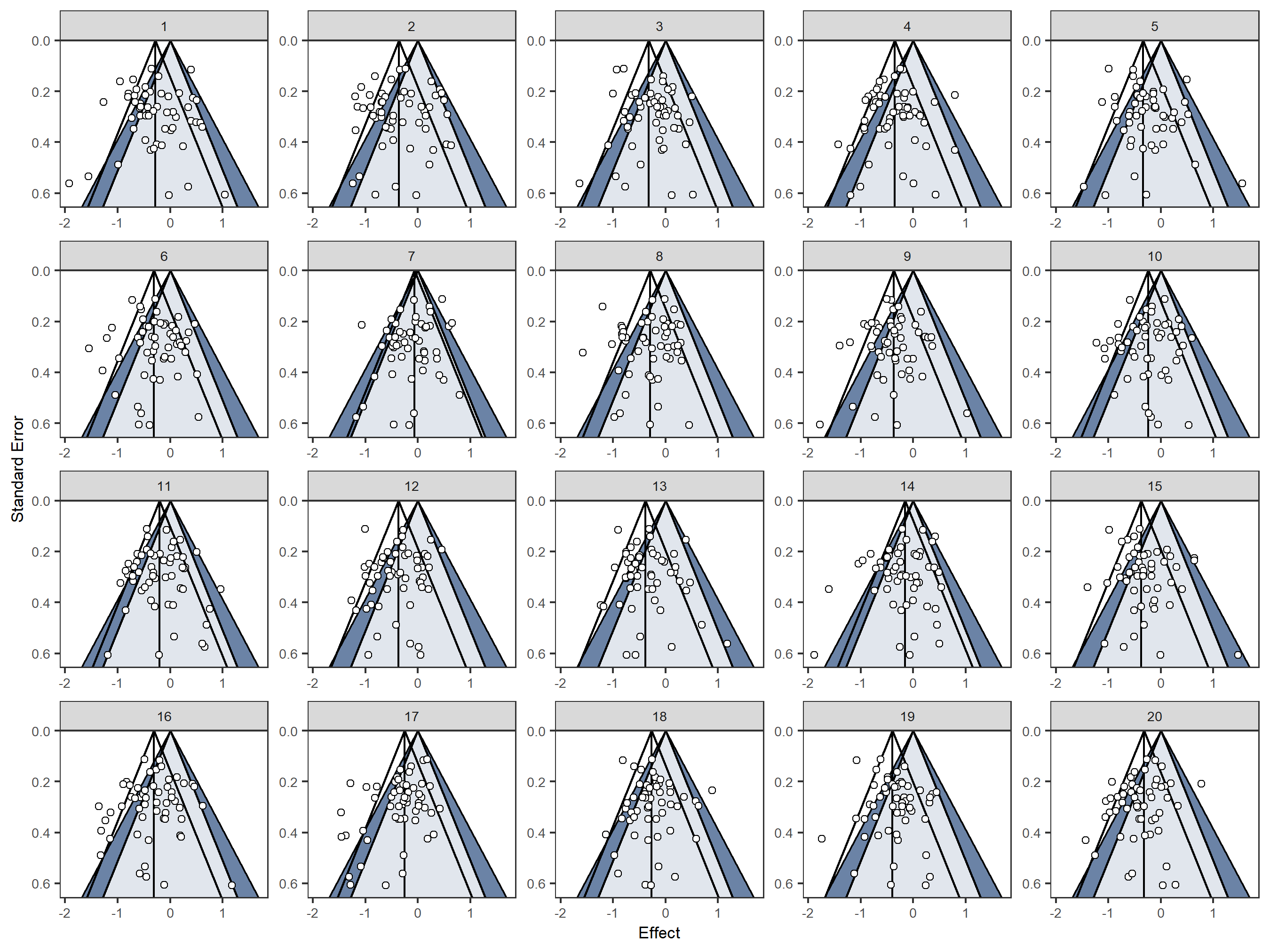To see the correct position of the real-data funnel plot after inspecting the lineup, the message printed in the R console can be run:

nullabor::decrypt("EwkU v7d7 9S YTV9d9TS Wo")
##  "True data in position  2"

Alternatively, a random seed can be set before creating the lineup. Then, after inspecting the lineup, the same lineup can be created (using the same random seed) but with the solution highlighted using the option show_solution = TRUE:

# set.seed(2)
# funnelinf(homeopath[, c("d", "se")])

set.seed(2) # same random seed as used above
funnelinf(homeopath[, c("d", "se")],
show_solution = TRUE)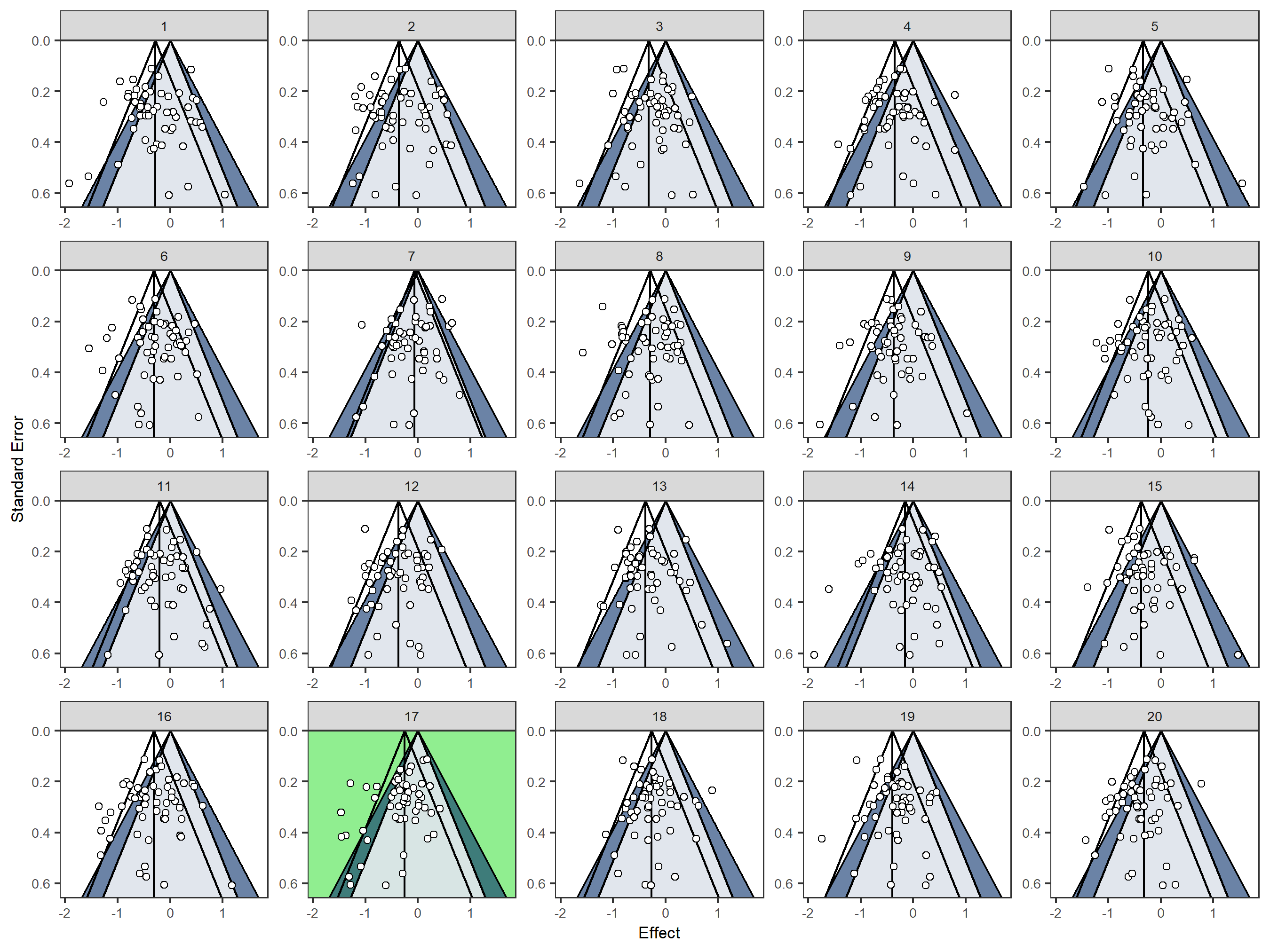Key features for creating the lineup and plotting the funnel graphs are presented below.

The most important lineup options are a) the model used for null plot simulation corresponding to the null hypothesis one seeks to reject and b) the number of plots used in the lineup corresponding to alpha level of the procedure.

A smaller lineup with only four plots (and therefore the alpha level controlled at 25%) is created using the option n

funnelinf(homeopath[, c("d", "se")],
n = 4)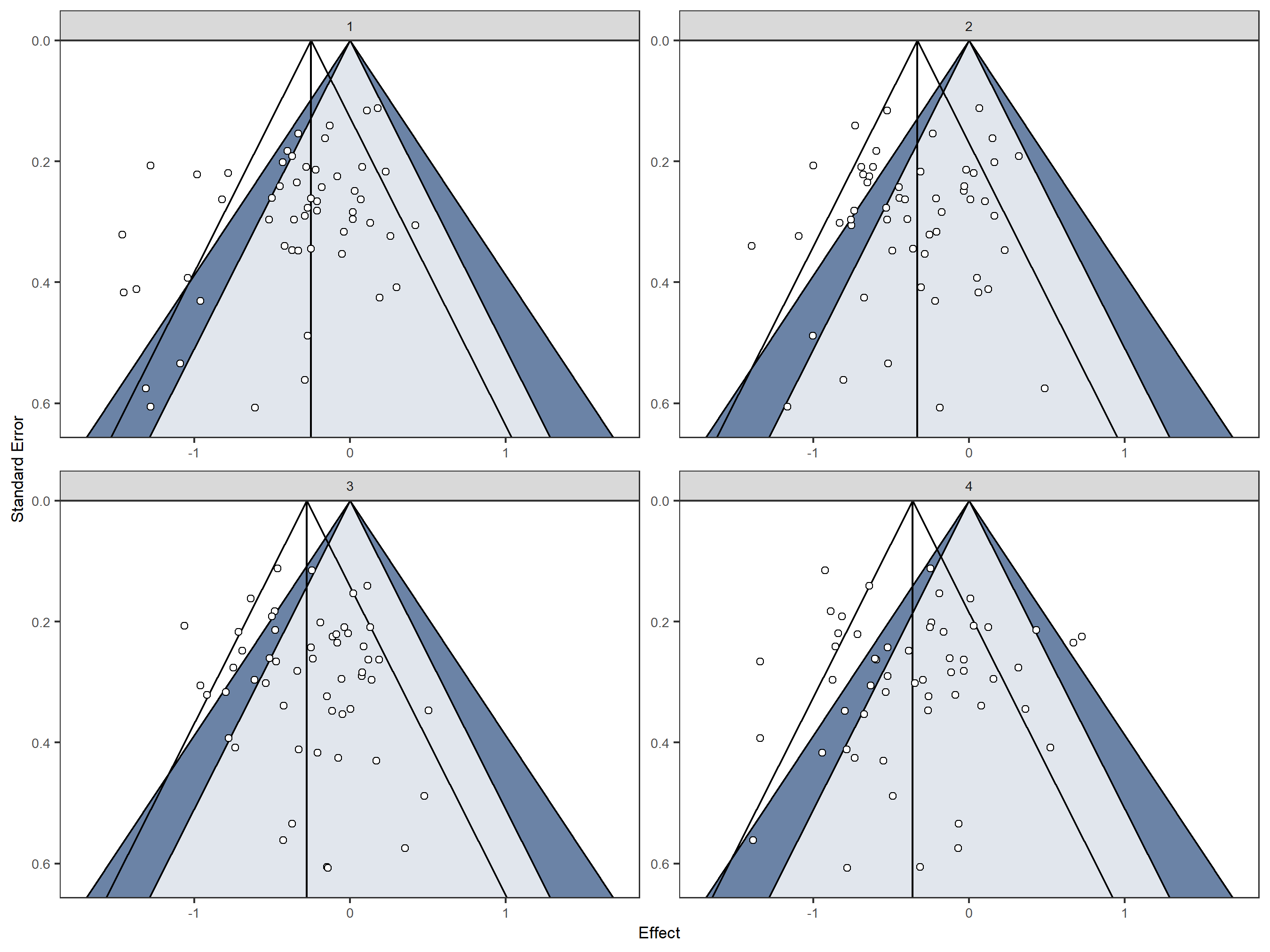The real-data funnel plot in this lineup can be found at position:

nullabor::decrypt("EwkU v7d7 9S YTV9d9TS Wu")
##  "True data in position  4"

Currently two widely used meta-analytic models are available to simulate data for the null funnel plots: the fixed effect and the random effects model. For details see Kossmeier, Tran, & Voracek (2019).

By default the random effects model is used for null plot simulation. Thus a potential excess between study variance (heterogeneity) observed in the real data is also incorporated in the null plots. If the presence of heterogeneity itself should be (at least partly) subject to visual inference, than the fixed effect model can be used by setting null_model = "FEM".

funnelinf(homeopath[, c("d", "se")], n = 4,
null_model = "FEM")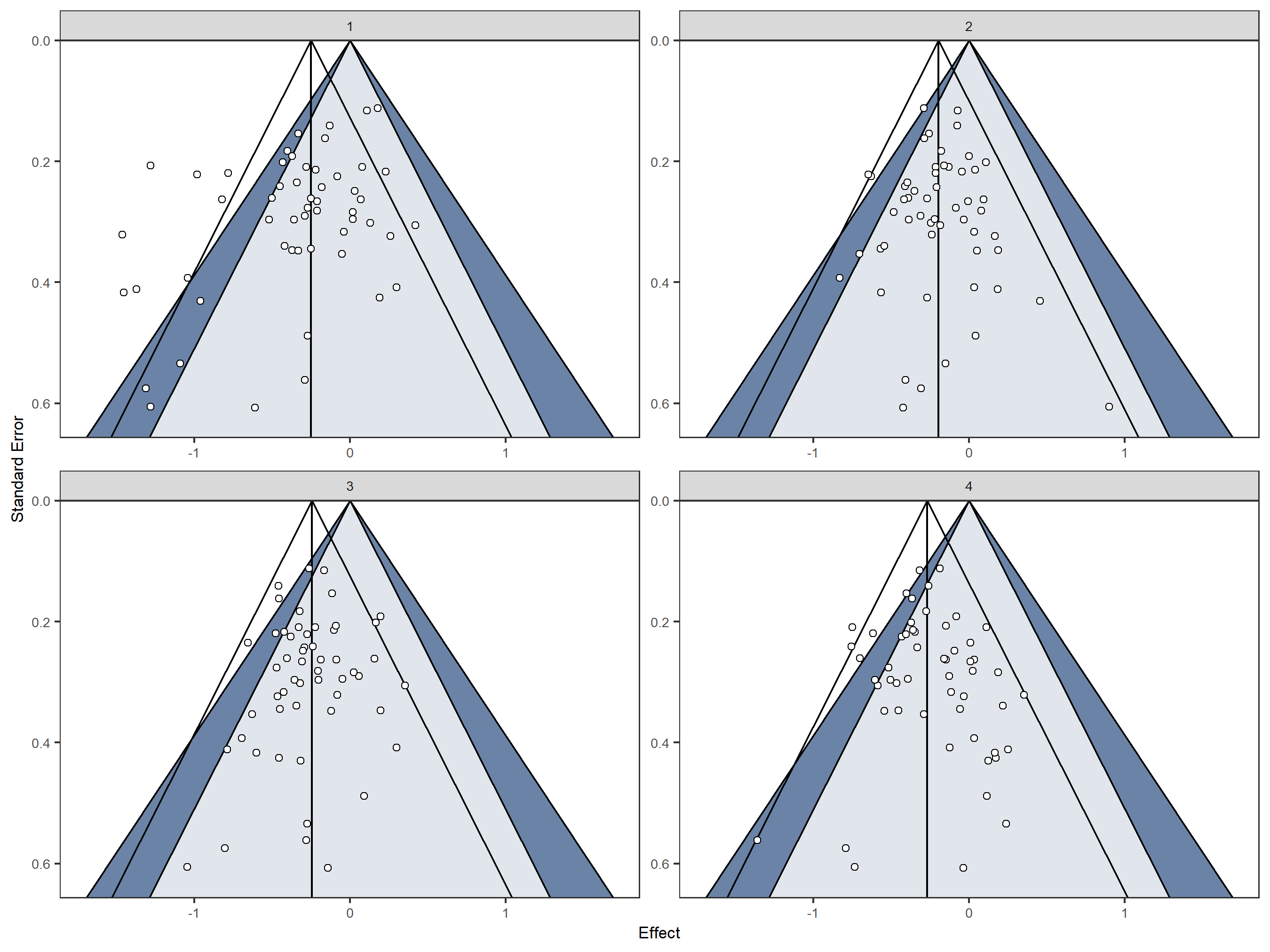The real-data funnel plot in this lineup can be found at position:

nullabor::decrypt("EwkU v7d7 9S YTV9d9TS WW")
##  "True data in position  1"

For training or educational purposes lineups entirely consisting of null funnel plots showing data simulated under the null hypothesis can be useful to get a feeling for which patterns are plausible under the null model (Buja et al. 2009). The parameters for simulating the null data are taken from the supplied real data but only null data plots are shown. Lineups entirely consisting of null funnel plots can be created with the option rorschach = TRUE

funnelinf(homeopath[, c("d", "se")], n = 4, null_model = "FEM",
rorschach = TRUE)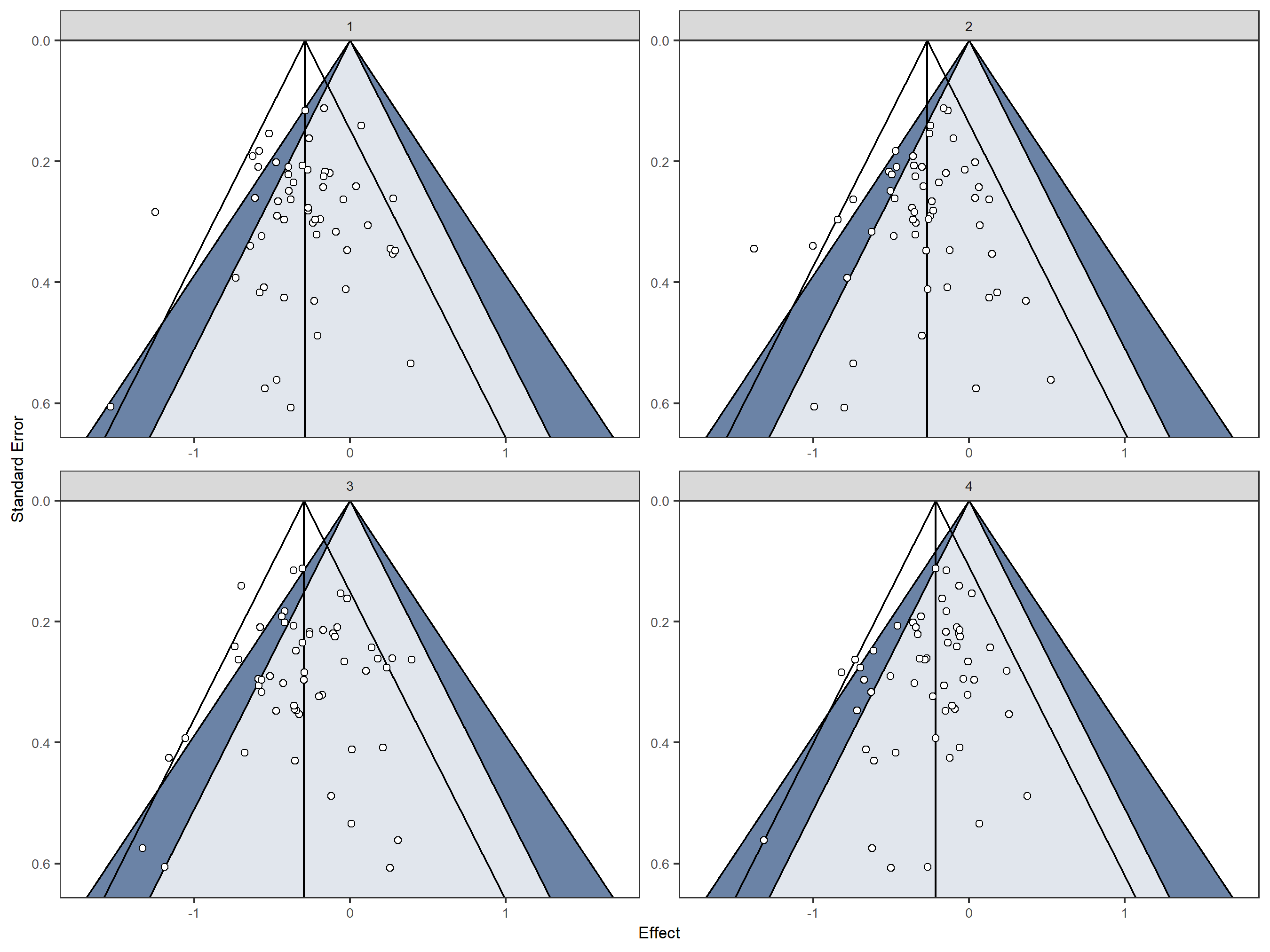## Funnel plot options

Several funnel plot variants can be used for visual funnel plot inference.

### Contours, axis

By default confidence (contours = TRUE) and significance contours (sig_contours = TRUE) are shown. In addition, instead of the standard error, the precision (i.e., 1 divided by the standard error) can be used on the y axis. This y-axis choice might have advantages if one or few large studies (with high precision) should be compared to the results of smaller studies condensed at the bottom of the funnel plot (Sterne & Egger, 2001).

funnelinf(homeopath[, c("d", "se")], n = 4,
y_axis = "precision")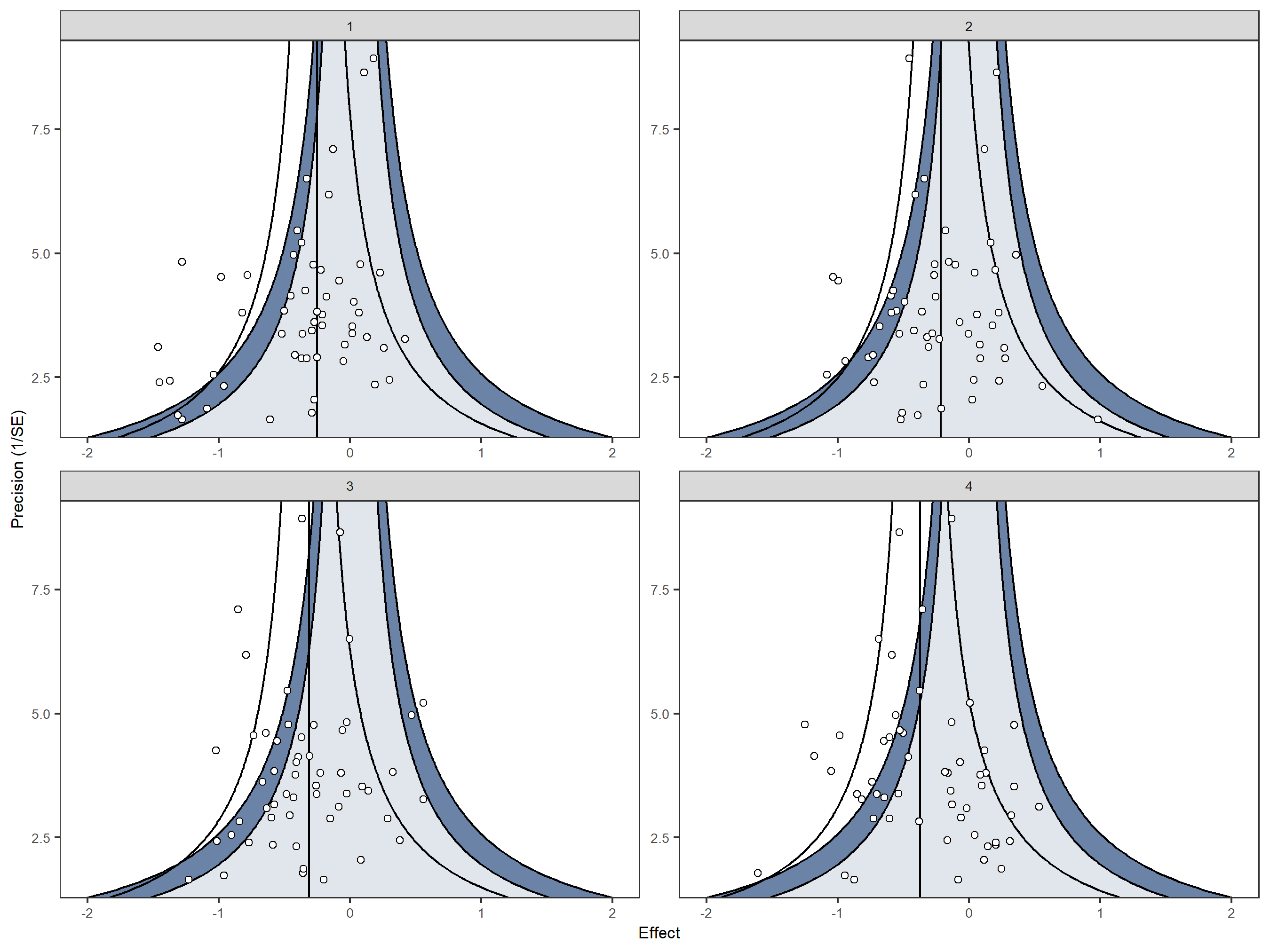### Trim-and-fill and Egger’s regression

The trim-and-fill algorithm (Duval & Tweedie, 2000), and Egger’s regression test (Egger, Smith, Schneider, & Minder, 1997) are two widely used methods to examine small study effects. Computing and showing their results in each funnel plot in the lineup might have the potential to increase the power of the lineup procedure to detect small-study effects. With egger = TRUE Egger’s regression line is drawn in each funnel plot and with trim_and_fill = TRUE imputed studies potentially missing due to publication bias are shown by black symbols. In the homeopath data used, small values (on the left-hand side of the funnel plot) are in favor of homeopathic treatment compared to placebo. Therefore studies potentially missing due to publication bias should be imputed on the right-hand side of the funnel plot by the trim-and-fill algorithm. This must be specified with the option trim_and_fill_side = "right".

funnelinf(homeopath[, c("d", "se")], n = 4,
egger = TRUE,
trim_and_fill = TRUE,
trim_and_fill_side = "right")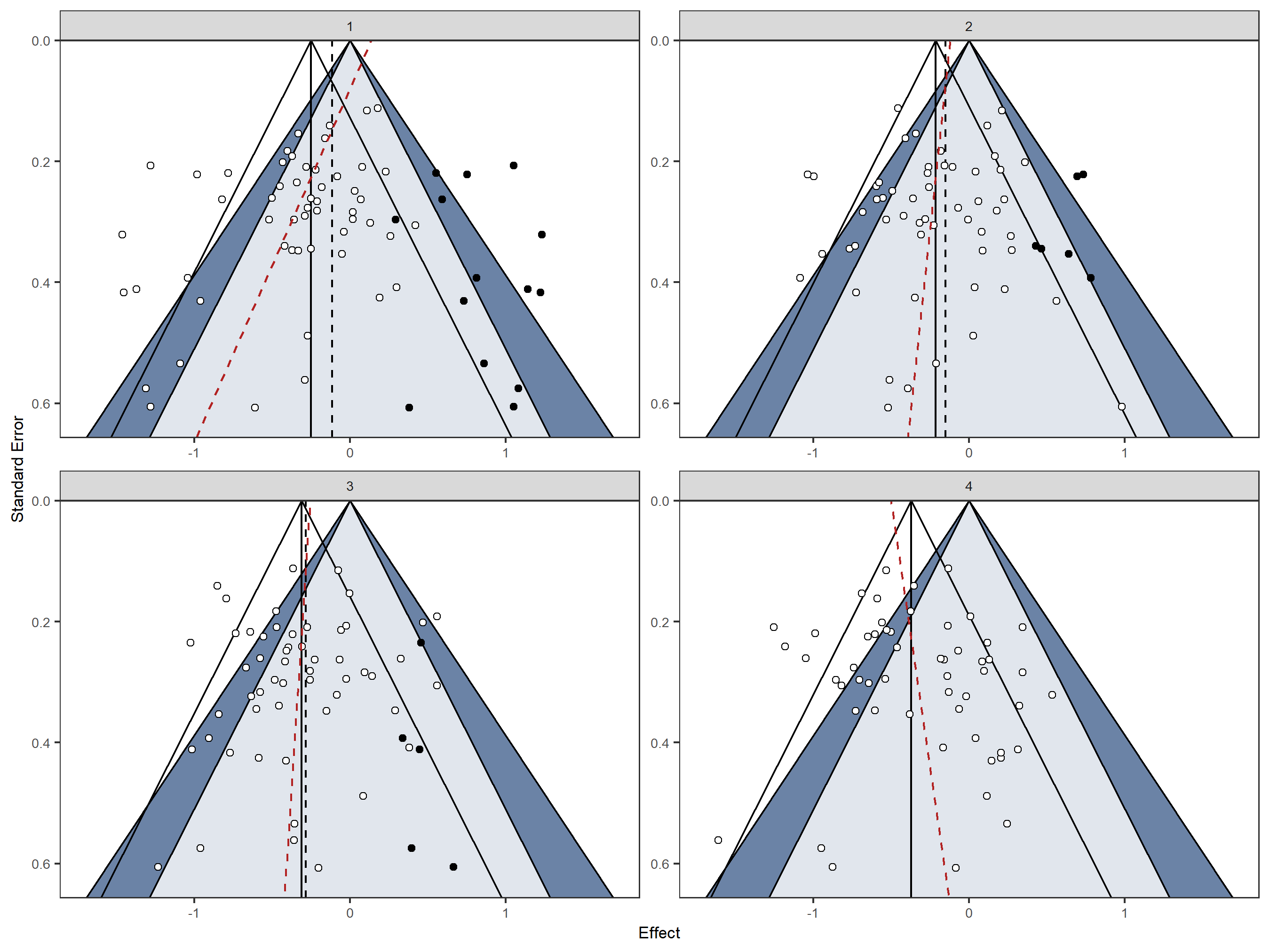### Subgroups

Studies can be plotted using different symbols with respect to their subgroup membership. For this purpose, a column with data on subgroup membership has to be specified.

First, we create a grouping variable for the homeopath data by dividing studies into older (publication year 2000 or earlier) and newer studies (publication year after 2000).

age <- factor(homeopath\$year > 2000, levels = c(FALSE, TRUE), labels = c("old", "new"))

This grouping information can now be incorporated into visual inference with funnel plots:

set.seed(4)
funnelinf(homeopath[, c("d", "se")], n = 4, null_model = "FEM",
group = age)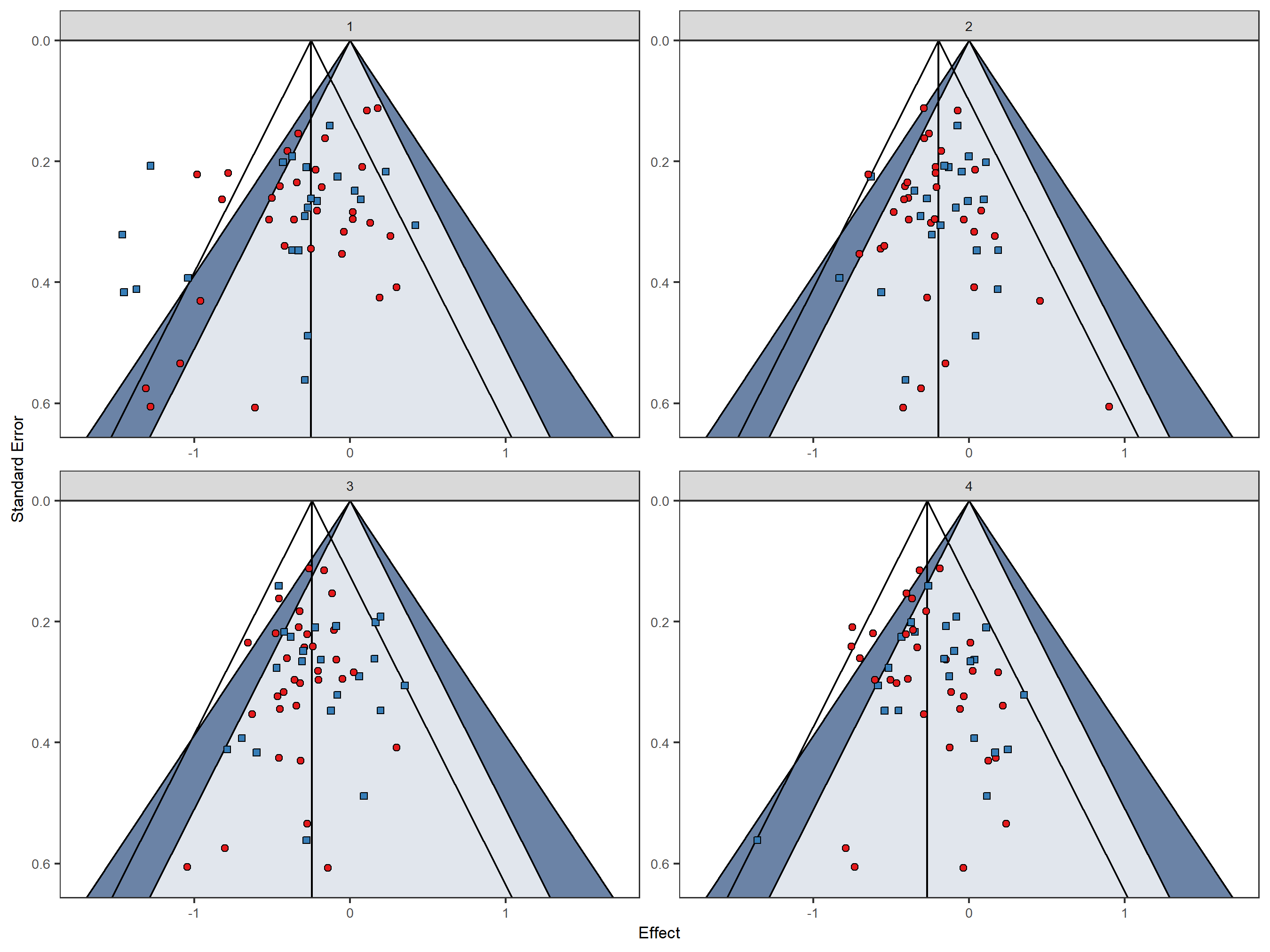## Contact

Questions, ideas, criticism: michael.kossmeier@univie.ac.at.